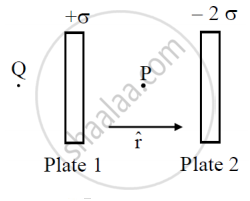# Two Parallel Uniformly Charged Infinite Plane Sheets, '1' and '2', Have Charge Densities + σ and − 2 σ Respectively. Give the Magnitude and Direction of the Net Electric Field at a Point. - Physics

Two parallel uniformly charged infinite plane sheets, '1' and '2', have charge densities + sigma and -2sigma respectively. Give the magnitude and direction of the net electric field at a point.
(i) in between the two sheets and
(ii) outside near the sheet '1'.

#### Solution

Which is same in both the case so it is independent of the size and shape of the closed surfaceLet hat6 be the unit vector directed from left to right

Let P and Q are two points in the inner and outer region of two plates respectively charge densities on plates are + sigma  and  -2sigma

(i) Electric field at point P in the inner region of the plates

vec"E"_1 = sigma/(2epsilon_0) hat"r" and vec"E"_2 = (2sigma)/(2epsilon_0)hat"r"

Net electric field in the inner region of the plates (i.e., at P) is

vec"E" = vec"E"_1 vec"E"_2

vec"E" = (sigma/(2epsilon_0)+ sigma/epsilon_0)hat"r"

vec"E" = (3sigma)/(2epsilon_0)hat"r"

(ii) Electric field at point Q in the outer region of plate 1

vec"E"_1 = sigma/(2epsilon_0)(-hat"r") and vec"E"_2 = (2sigma)/(2epsilon_0)hat"r"

Net electric field in the outer region of plate 1 (i.e. at Q ) is

vec"E" = vec"E"_1 + vec"E"_2 = (sigma/epsilon_0 - sigma/(2epsilon_0))hat"r"

vec"E" = sigma/(2epsilon_0)hat"r"

Concept: Capacitance of a Parallel Plate Capacitor with and Without Dielectric Medium Between the Plates
Is there an error in this question or solution?